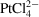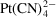International Tables for Crystallography (2006). Vol. F, ch. 12.1, pp. 247-255   | 1 | 2 | https://doi.org/10.1107/97809553602060000679

Contents

• 12.1. The preparation of heavy-atom derivatives of protein crystals for use in multiple isomorphous replacement and anomalous scattering  (pp. 247-255)
• 12.1.1. Introduction  (p. 247) | html | pdf |
• 12.1.2. Heavy-atom data bank  (pp. 247-248) | html | pdf |
• 12.1.3. Properties of heavy-atom compounds and their complexes  (pp. 248-250) | html | pdf |
• 12.1.3.1. Stability  (p. 248) | html | pdf |
• 12.1.3.2. Lability  (p. 248) | html | pdf |
• 12.1.3.3. Oxidation state of metal ions in protein crystals  (p. 248) | html | pdf |
• 12.1.3.4. Effect of pH  (pp. 248-249) | html | pdf |
• 12.1.3.5. Effect of precipitants and buffers on heavy-atom binding  (p. 249) | html | pdf |
• 12.1.3.6. Solubility of heavy-atom compounds  (p. 249) | html | pdf |
• 12.1.3.7. Effect of concentration, time of soak and temperature on heavy-atom binding  (p. 250) | html | pdf |
• 12.1.4. Amino acids as ligands  (p. 250) | html | pdf |
• 12.1.5. Protein chemistry of heavy-atom reagents  (pp. 250-254) | html | pdf |
• 12.1.5.1. Hard cations  (p. 251) | html | pdf |
• 12.1.5.2. Thallium and lead ions  (p. 251) | html | pdf |
• 12.1.5.3. B-metal reagents  (pp. 251-253) | html | pdf |
• 12.1.5.4. Electrostatic binding of heavy-atom anions  (p. 253) | html | pdf |
• 12.1.5.5. Hydrophobic heavy-atom reagents  (pp. 253-254) | html | pdf |
• 12.1.5.6. Iodine  (p. 254) | html | pdf |
• 12.1.5.7. Polynuclear reagents  (p. 254) | html | pdf |
• 12.1.6. Metal-ion replacement in metalloproteins  (pp. 254-255) | html | pdf |
• 12.1.7. Analogues of amino acids  (p. 255) | html | pdf |
• 12.1.8. Use of the heavy-atom data bank to select derivatives  (p. 255) | html | pdf |
• References | html | pdf |
• Figures
• Fig. 12.1.5.1. The binding site for uranyl ions in cytochrome b 5 (oxidized: 3B5C)  (p. 252) | html | pdf |
• Fig. 12.1.5.2. Mercuric ions replace zinc in thermolysin (3TLN)  (p. 252) | html | pdf |
• Fig. 12.1.5.3. The binding of a silver ion to immunoglobulin Fab (2FB4)  (p. 252) | html | pdf |
• Fig. 12.1.5.4. The binding ofthrough a methionine in azurin (1AZU)  (p. 252) | html | pdf |
• Fig. 12.1.5.5. The relative positions of methionine side chains (carbon: green; sulfur: yellow) in the parent crystals to the binding of platinum (pink) of(p. 252) | html | pdf |
• Fig. 12.1.5.6. The relative positions of cystine disulfide bridges (carbon: green; sulfur: yellow) in the parent crystals to the binding of platinum (pink) of(p. 253) | html | pdf |
• Fig. 12.1.5.7. The binding ofto aldose dehydrogenase (8ADH)  (p. 253) | html | pdf |
• Fig. 12.1.6.1. The displacement of calcium by samarium in thermolysin  (p. 254) | html | pdf |
• Tables
• Table 12.1.3.1. Useful pH ranges of some heavy-atom reagents derived from the heavy-atom data bank  (p. 249) | html | pdf |
• Table 12.1.5.1. The 23 most commonly used heavy-atom reagents  (p. 251) | html | pdf |
• Table 12.1.5.2. The five most popular uranium derivatives  (p. 251) | html | pdf |
• Table 12.1.5.3. The five most popular mercury derivatives  (p. 251) | html | pdf |
• Table 12.1.5.4. The five most popular platinum derivatives  (p. 253) | html | pdf |